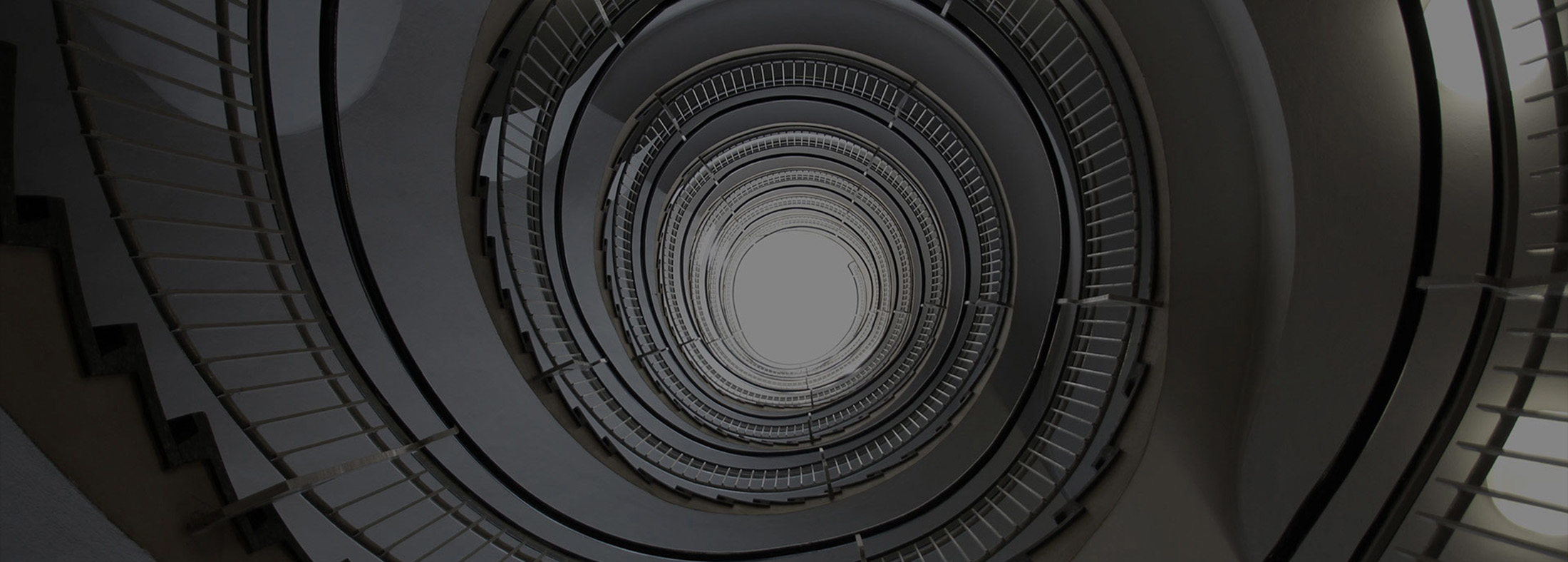# Secondary 3 A Maths

##### O Level A Math Tuition

Conquer the rigour of additional mathematics with our innovative lessons.

## Our Lesson Syllabus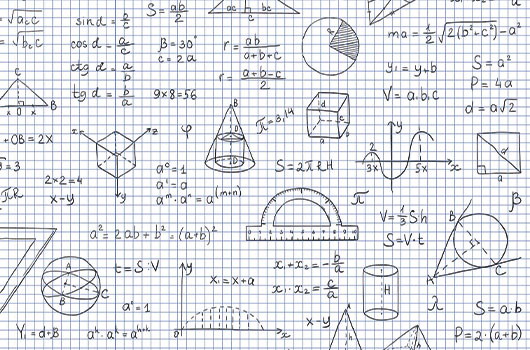### Term 1

2. Remainder and Factor Theorem

3. Partial Fractions

4. Indices and Surds

5. Exponentials and Logarithms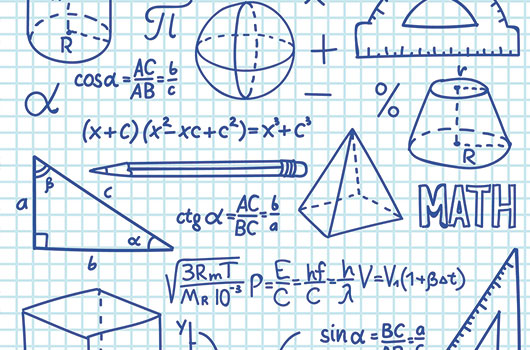### Term 2

1. Linear Law

2. Coordinate Geometry

3. Further Coordinate Geometry

4. Modulus Function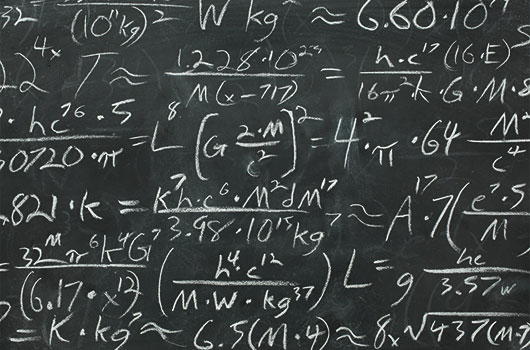### Term 3

1. Binomial Theorem

2. Trigonometric Functions and Graphs

3. Compound Angle and
R-Formulae

4. Trigonometric Identities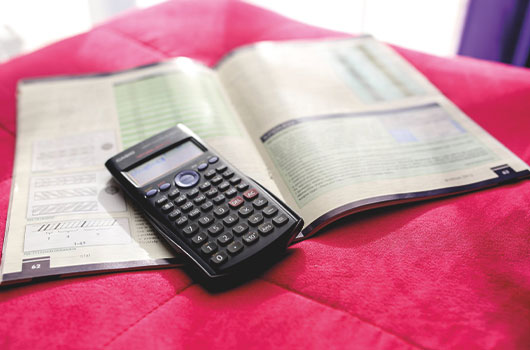### Term 4

1. End-of-Year Preparation

Need Help? Chat with us